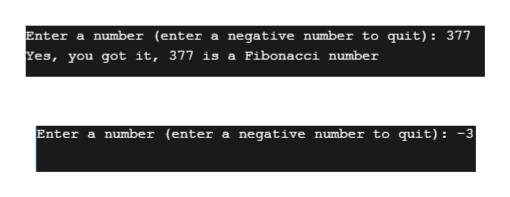# C++ can someone help me with this code?The code doesnt run, says there an error at exit(0) and can someone explain in detail how it is using recursive to find the FIB number ..#include <iostream>using namespace std;//main methodint main(){    //variable declaration        int num, num1 = 0, num2 = 1, temp;        // getting input from user       cout<<"Enter a number (enter a negative number to quit): ";        cin>>num;        //if the number entered by user is negative then exit the program         if (num<0)        {            //exit statement            exit(0);        }        // 0 and 1 are fibonacci numbers        if (num1==num || num2==num)        {                cout<<"%d is a fibonacci number\n"<< num;                return 0;        }         // checking whether a given number is Fibonacci or not        while (num2 <= num)        {            //assigning the value of second number to temp variable                temp = num2;                //assigning the sum value of num1 and num2 to num2                num2 = num1 + num2;                //assigning temp varible value to num1                num1 = temp;                //if num2 is equal to the number entered by user                if (num2 == num)                {                        break;                }        }        // printing the results        if (num1==num||num2==num)        {            //displaying message to user that entered number is a fibonacci number           cout<<"Yes, you got it, " <<num<< " is a Fibonacci number";              }        else        {            //displaying message to user that the entered number is not a fibonacci number                cout<<"!!!!! Sorry "<<num <<" is not a Fibonacci number";        }        return 0;  }

Question
7 views

C++ can someone help me with this code?

The code doesnt run, says there an error at exit(0)

and can someone explain in detail how it is using recursive to find the FIB number ..

#include <iostream>

using namespace std;

//main method

int main()

{

//variable declaration

int num, num1 = 0, num2 = 1, temp;

// getting input from user

cout<<"Enter a number (enter a negative number to quit): ";

cin>>num;

//if the number entered by user is negative then exit the program

if (num<0)

{

//exit statement

exit(0);

}

// 0 and 1 are fibonacci numbers

if (num1==num || num2==num)

{

cout<<"%d is a fibonacci number\n"<< num;

return 0;

}

// checking whether a given number is Fibonacci or not

while (num2 <= num)

{

//assigning the value of second number to temp variable

temp = num2;

//assigning the sum value of num1 and num2 to num2

num2 = num1 + num2;

//assigning temp varible value to num1

num1 = temp;

//if num2 is equal to the number entered by user

if (num2 == num)

{

break;

}

}

// printing the results

if (num1==num||num2==num)

{

//displaying message to user that entered number is a fibonacci number

cout<<"Yes, you got it, " <<num<< " is a Fibonacci number";

}

else

{

//displaying message to user that the entered number is not a fibonacci number

cout<<"!!!!! Sorry "<<num <<" is not a Fibonacci number";

}

return 0;

}

check_circle

star
star
star
star
star
1 Rating
Step 1

The exit() function is used to end the normal termination of a program. In c++ It is defined in the cstdlib header file. To use this use header file #include<cstdlib>

The exit(0) function returns a value of 0 to the IDE showing that the program reached the normal termination. It indicated the successful termination of the program.

The exit(1) function is used to indicate that the program will exit due to some error condition. It indicated unsuccessful termination of the program.

Step 2

In the Fibonacci series, the next number is the sum of the first two preceding numbers.

The Fibonacci series is started at 0, and the next number is 1.

The third number is the sum of these two numbers means 0 + 1 = 1, hence the third number is also 1.

The fourth number is the sum of the second and the third number means 1 + 1 = 2, hence the fourth number is 2.

Therefore, the Fibonacci series is:

0, 1, 1, 2, 3, 5, 8, 13, 21, 34, 55, 89, 144, 233, 377, 610, 987………..

Step 3

The program is:

#include <iostream>

#include <cstdlib>

using namespace std;

//main method

int main()

{

//variable declaration

int num, num1 = 0, num2 = 1, temp;

// getting input from user

cout<<"Enter a number (enter a negative number to quit): ";

cin>>num;

//If the number entered by the user is negative then exit the program

if (num<0)

{

//exit statement

exit(0);

}

// 0 and 1 are fibonacci numbers

if (num1==num || num2==num)

{

cout << num <<" is a fibonacci number\n";

return 0;

}

// checking whether a given number is Fibonacci or not

while (num2 <= num)

{

//assigning the value of the second number to a temp variable

temp = num2;

//assigning the sum value of num1 and num2 to num2

num2 = num1 + num2;

//assigning temp varible value to num1

...help_outlineImage TranscriptioncloseEnter a number (enter a negative number to quit): 377 Yes, you got it, 377 is a Fibonacci number Enter a number (enter a negative number to quit): -3 fullscreen

### Want to see the full answer?

See Solution

#### Want to see this answer and more?

Solutions are written by subject experts who are available 24/7. Questions are typically answered within 1 hour.*

See Solution
*Response times may vary by subject and question.
Tagged in

### Computer Science